# Forces On An Inclined Surface

This topic is part of the HSC Physics course under the section Forces, Acceleration and Energy.

### HSC Physics Syllabus

• explore the concept of net force and equilibrium in one-dimensional and simple two-dimensional contexts using: (ACSPH050)

– vector addition by resolution into components
• solve problems or make quantitative predictions about resultant and component forces by applying the following relationships:

– F_{AB} = -F_{BA}

– F_x=Fcosθ, F_y=Fsinθ

• conduct a practical investigation to explain and predict the motion of objects on inclined planes (ACSPH098)

### Forces on an Inclined Surface WITH Friction

Forces on an inclined surface is a crucial topic to grasp in HSC Physics as it forms the basis for understanding various real-world applications such as ramps, inclined planes, and more.

### Forces on an Inclined Surface

When an object is placed on an inclined surface, it experiences various forces acting upon it.

The two primary forces at play are:

• Gravitational force (F_g): The force exerted by Earth on the object, which acts vertically downwards. This is also known as the weight force.
• Normal force (F_N): The perpendicular force exerted by the surface on the object, acting at a 90-degree angle to the surface.

### Resolving the Weight Force

To analyse the forces acting on the object, we need to resolve the gravitational force into two components:

• Perpendicular to the surface (F_{g⊥}): The component of gravitational force that acts in the opposite direction of the normal force.
• Parallel to the surface (F_{g‖}): The component of gravitational force that acts along the incline.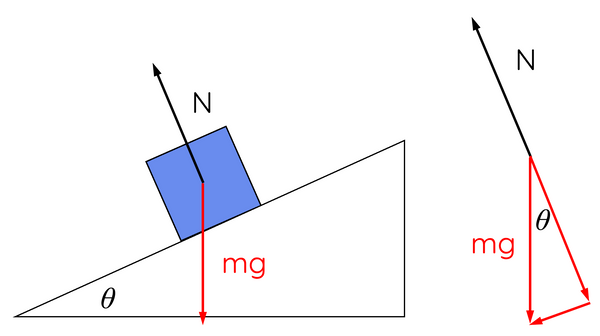Using trigonometry, we can derive the following relationships:

$$F_{g⊥} = F_g \times \cos{\theta}$$

$$F_{g‖} = F_g \times \sin{\theta}$$

Where θ is the angle between the inclined surface and the horizontal plane, and F_g = mg, with m representing the mass of the object and g being the acceleration due to gravity (approximately 9.81 m/s²).

### No Friction Scenario

In the absence of friction, the only force acting on the object along the incline is F_{g‖}. This force causes the object to accelerate down the incline, and the acceleration can be calculated using Newton's second law:

$$F_{net} = ma$$

$$F_{g‖} = ma$$

$$mg \times \sin{\theta} = ma$$

$$a = g \times \sin{\theta}$$

In this case, the acceleration of the object is independent of its mass and solely depends on the angle of the incline and the acceleration due to gravity. In the absence of friction, the parallel component of weight force is never balanced by any other forces, so the mass will also undergo motion with acceleration.

### With Friction Scenario

When friction is present, we need to consider the force of friction (F_f) acting on the object.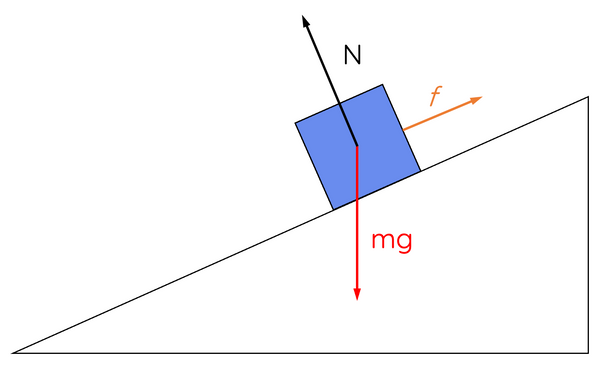The force of friction opposes the motion of the object and is proportional to the normal force acting on it:

$$F_f = μ \times F_n$$

Where μ is the coefficient of friction (a dimensionless value).

In this scenario, the net force acting on the object along the incline is given by:

$$F_{net} = F_{g‖} - F_f$$

Using Newton's second law, we can determine the acceleration:

$$ma = F_{g‖} - F_f$$

Since F_n = F_{g⊥}, we can rewrite the frictional force as:

$$F_f = \mu \times F_{g⊥}$$

$$F_f = \mu times mg \cos{\theta}$$

Now, we can find the acceleration:

$$a = \frac{F_{g‖} - F_f}{m}$$

$$a = \frac{mg \sin{\theta} - \mu mg \cos{\theta}}{m}$$

$$a = g \sin{\theta} - \mu \cos{\theta}$$

In this case, the acceleration of the object is dependent on the angle of the incline, the acceleration due to gravity, and the coefficient of friction.

When the parallel component of weight force acting down the incline is balanced by friction acting up the incline, the net force acting on the mass would equal zero. As a result, the object is in equilibrium (either at rest or moving at constant velocity).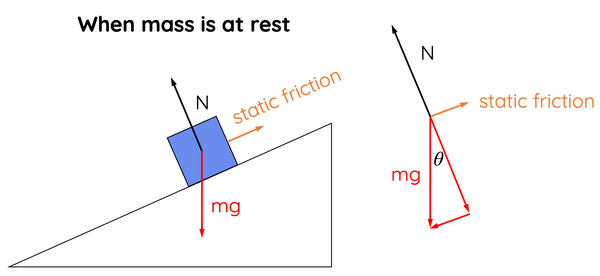When a mass is at rest on an inclined surface, the parallel component of weight force is balanced by static friction.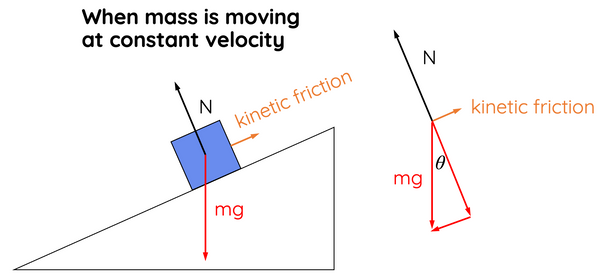When a mass is moving at constant velocity, the parallel component of weight force is balanced by kinetic friction.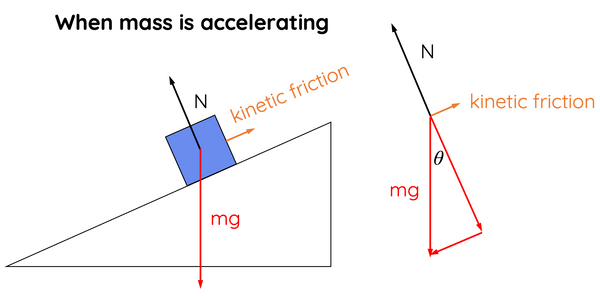When the parallel component of weight force is greater than the kinetic friction, the mass will accelerate down the incline.